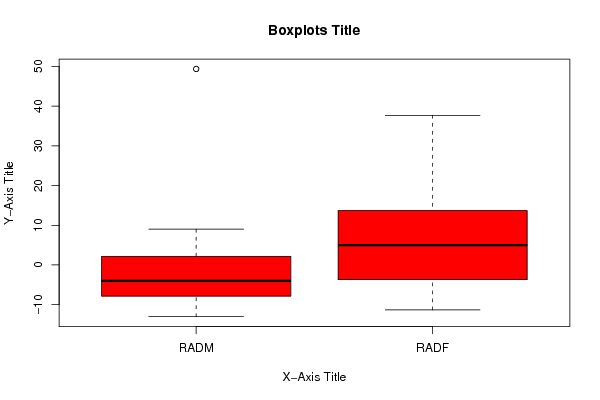## Free Statistics

of Irreproducible Research!

Author's title
Author*The author of this computation has been verified*
R Software ModuleIan.Hollidayrwasp_Tests to Compare Two Means.wasp
Title produced by softwareT-Tests
Date of computationSun, 29 May 2011 17:38:02 +0000
Cite this page as followsStatistical Computations at FreeStatistics.org, Office for Research Development and Education, URL https://freestatistics.org/blog/index.php?v=date/2011/May/29/t1306690537ffyqa517w8cxbdp.htm/, Retrieved Thu, 06 Oct 2022 13:27:22 +0000
Statistical Computations at FreeStatistics.org, Office for Research Development and Education, URL https://freestatistics.org/blog/index.php?pk=122557, Retrieved Thu, 06 Oct 2022 13:27:22 +0000
QR Codes:Original text written by user:
IsPrivate?No (this computation is public)
User-defined keywords
Estimated Impact154
Family? (F = Feedback message, R = changed R code, M = changed R Module, P = changed Parameters, D = changed Data)
-     [Aston University Statistical Software] [Morning Sickness ...] [2009-11-16 16:26:06] [74be16979710d4c4e7c6647856088456]
- R     [Aston University Statistical Software] [Morning Sickness ...] [2009-11-16 17:22:16] [74be16979710d4c4e7c6647856088456]
-   P     [T-Tests] [Morning Sickness ...] [2010-11-09 11:12:43] [3fdd735c61ad38cbc9b3393dc997cdb7]
-           [T-Tests] [Week 6 compendium...] [2010-11-12 14:20:50] [88bba52b01540967ecde38774018061d]
- R  D          [T-Tests] [t test] [2011-05-29 17:38:02] [add39d12cbc73ea169e24b6abf6153f7] [Current]
- RMPD            [Correlation] [correlation] [2011-05-29 18:08:41] [88bba52b01540967ecde38774018061d]
- R                 [Correlation] [spearman] [2011-05-29 18:11:55] [88bba52b01540967ecde38774018061d]
-                     [Correlation] [spearman] [2011-05-29 18:15:43] [88bba52b01540967ecde38774018061d]
-   PD            [T-Tests] [compare female] [2011-05-30 17:56:07] [88bba52b01540967ecde38774018061d]
Feedback Forum

Post a new message
Dataseries X:
-6.5	-2.67
-7.33	9.67
49.33	-6
-11	28.33
-8.33	12
9	-11.3
2.33	3
-12.3	-4.67
5.67	-5
-2	2.33
1.33	37.67
-13	7.5
-5.67	5
-4	30
2	15.33

 Summary of computational transaction Raw Input view raw input (R code) Raw Output view raw output of R engine Computing time 1 seconds R Server 'Herman Ole Andreas Wold' @ www.yougetit.org

\begin{tabular}{lllllllll}
\hline
Summary of computational transaction \tabularnewline
Raw Input & view raw input (R code)  \tabularnewline
Raw Output & view raw output of R engine  \tabularnewline
Computing time & 1 seconds \tabularnewline
R Server & 'Herman Ole Andreas Wold' @ www.yougetit.org \tabularnewline
\hline
\end{tabular}
%Source: https://freestatistics.org/blog/index.php?pk=122557&T=0

[TABLE]
[ROW][C]Summary of computational transaction[/C][/ROW]
[ROW][C]Raw Input[/C][C]view raw input (R code) [/C][/ROW]
[ROW][C]Raw Output[/C][C]view raw output of R engine [/C][/ROW]
[ROW][C]Computing time[/C][C]1 seconds[/C][/ROW]
[ROW][C]R Server[/C][C]'Herman Ole Andreas Wold' @ www.yougetit.org[/C][/ROW]
[/TABLE]
Source: https://freestatistics.org/blog/index.php?pk=122557&T=0

Globally Unique Identifier (entire table): ba.freestatistics.org/blog/index.php?pk=122557&T=0

As an alternative you can also use a QR Code:

The GUIDs for individual cells are displayed in the table below:

 Summary of computational transaction Raw Input view raw input (R code) Raw Output view raw output of R engine Computing time 1 seconds R Server 'Herman Ole Andreas Wold' @ www.yougetit.org

 T-Test Mean1 -0.03133 Mean2 8.07933 T Statistic -1.49937 P-value 0.14499 Lower Confidence Limit -19.19238 Upper Confidence Limit 2.97105

\begin{tabular}{lllllllll}
\hline
T-Test \tabularnewline
Mean1 & -0.03133 \tabularnewline
Mean2 & 8.07933 \tabularnewline
T Statistic & -1.49937 \tabularnewline
P-value & 0.14499 \tabularnewline
Lower Confidence Limit & -19.19238 \tabularnewline
Upper Confidence Limit & 2.97105 \tabularnewline
\hline
\end{tabular}
%Source: https://freestatistics.org/blog/index.php?pk=122557&T=1

[TABLE]
[ROW][C]T-Test[/C][/ROW]
[ROW][C]Mean1[/C][C]-0.03133[/C][/ROW]
[ROW][C]Mean2[/C][C]8.07933[/C][/ROW]
[ROW][C]T Statistic[/C][C]-1.49937[/C][/ROW]
[ROW][C]P-value[/C][C]0.14499[/C][/ROW]
[ROW][C]Lower Confidence Limit[/C][C]-19.19238[/C][/ROW]
[ROW][C]Upper Confidence Limit[/C][C]2.97105[/C][/ROW]
[/TABLE]
Source: https://freestatistics.org/blog/index.php?pk=122557&T=1

Globally Unique Identifier (entire table): ba.freestatistics.org/blog/index.php?pk=122557&T=1

As an alternative you can also use a QR Code:

The GUIDs for individual cells are displayed in the table below:

 T-Test Mean1 -0.03133 Mean2 8.07933 T Statistic -1.49937 P-value 0.14499 Lower Confidence Limit -19.19238 Upper Confidence Limit 2.97105

 Standard Deviations Variable 1 15.15771 Variable 2 14.46253

\begin{tabular}{lllllllll}
\hline
Standard Deviations \tabularnewline
Variable 1 & 15.15771 \tabularnewline
Variable 2 & 14.46253 \tabularnewline
\hline
\end{tabular}
%Source: https://freestatistics.org/blog/index.php?pk=122557&T=2

[TABLE]
[ROW][C]Standard Deviations[/C][/ROW]
[ROW][C]Variable 1[/C][C]15.15771[/C][/ROW]
[ROW][C]Variable 2[/C][C]14.46253[/C][/ROW]
[/TABLE]
Source: https://freestatistics.org/blog/index.php?pk=122557&T=2

Globally Unique Identifier (entire table): ba.freestatistics.org/blog/index.php?pk=122557&T=2

As an alternative you can also use a QR Code:

The GUIDs for individual cells are displayed in the table below:

 Standard Deviations Variable 1 15.15771 Variable 2 14.46253PNG link Postscript link PDF link

Parameters (Session):
par1 = two.sided ; par2 = 1 ; par3 = 2 ; par4 = T-Test ; par5 = unpaired ; par6 = 0.0 ; par7 = .95 ; par8 = TRUE ;
Parameters (R input):
par1 = two.sided ; par2 = 1 ; par3 = 2 ; par4 = T-Test ; par5 = unpaired ; par6 = 0.0 ; par7 = .95 ; par8 = TRUE ;
R code (references can be found in the software module):
par2 <- as.numeric(par2)par3 <- as.numeric(par3)par4 <- as.character(par4)par5 <- as.character(par5)par6 <- as.numeric(par6)par7 <- as.numeric(par7)par8 <- as.logical(par8)if ( par5 == 'unpaired') paired <- FALSE else paired <- TRUEx <- t(y)if(par8){bitmap(file='test1.png')(r<-boxplot(x ,xlab=xlab,ylab=ylab,main=main,notch=FALSE,col=2))dev.off()}load(file='createtable')if( par4 == 'Wilcoxon-Mann_Whitney'){a<-table.start()a <- table.row.start(a)a <- table.element(a,'Wilcoxon Test',3,TRUE)a <- table.row.end(a)a <- table.row.start(a)a <- table.element(a,'',1,TRUE)a <- table.element(a,'Statistic',1,TRUE)a <- table.element(a,'P-value',1,TRUE)a <- table.row.end(a)W <- wilcox.test(x[,par2],x[,par3],alternative=par1, paired = paired)a<-table.row.start(a)a<-table.element(a,'Wilcoxon Test',1,TRUE)a<-table.element(a,W$statistic[])a<-table.element(a,round(W$p.value, digits=5) )a<-table.row.end(a)a<-table.end(a)table.save(a,file='mytable.tab')}if( par4 == 'T-Test'){T <- t.test(x[,par2],x[,par3],alternative=par1, paired=paired, mu=par6, conf.level=par7)a<-table.start()a <- table.row.start(a)a <- table.element(a,'T-Test',3,TRUE)a <- table.row.end(a)if(paired){a <- table.row.start(a)a <- table.element(a,'Difference: Mean1 - Mean2',1,TRUE)a<-table.element(a,round(T$estimate, digits=5) )a <- table.row.end(a)}if(!paired){a <- table.row.start(a)a <- table.element(a,'Mean1',1,TRUE)a<-table.element(a,round(T$estimate, digits=5) )a <- table.row.end(a)a <- table.row.start(a)a <- table.element(a,'Mean2',1,TRUE)a<-table.element(a,round(T$estimate, digits=5) )a <- table.row.end(a)}a <- table.row.start(a)a <- table.element(a,'T Statistic',1,TRUE)a<-table.element(a,round(T$statistic, digits=5) )a <- table.row.end(a)a <- table.row.start(a)a <- table.element(a,'P-value',1,TRUE)a<-table.element(a,round(T$p.value, digits=5) )a <- table.row.end(a)a <- table.row.start(a)a <- table.element(a,'Lower Confidence Limit',1,TRUE)a<-table.element(a,round(T$conf.int, digits=5) )a <- table.row.end(a)a<-table.row.start(a)a <- table.element(a,'Upper Confidence Limit',1,TRUE)a<-table.element(a,round(T\$conf.int, digits=5) )a <- table.row.end(a)a<-table.end(a)table.save(a,file='mytable.tab')}a<-table.start()a <- table.row.start(a)a <- table.element(a,'Standard Deviations',3,TRUE)a <- table.row.end(a)a <- table.row.start(a)a <- table.element(a,'Variable 1',1,TRUE)a<-table.element(a,round(sd(x[,par2]), digits=5) )a <- table.row.end(a)a <- table.row.start(a)a <- table.element(a,'Variable 2',1,TRUE)a<-table.element(a,round(sd(x[,par3]), digits=5) )a <- table.row.end(a)a<-table.end(a)table.save(a,file='mytable1.tab')